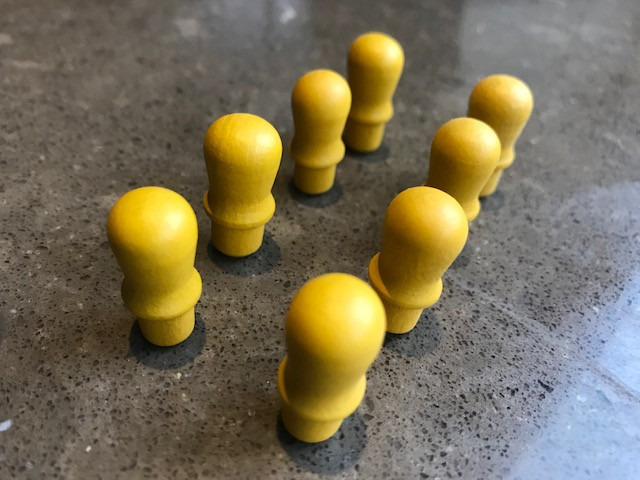Search

# The power of doublingBeing able to quickly double numbers is great for addition as well as the two times table.

As with all multiplication problems, if you know how to work out the answers, that is great. But if you "just know" the answers because you have learned them off by heart, your working memory will be freed up to deal with the next level of numerical challenge.A child who can recall the doubles of single numbers can use this skill to help with so much more:

Near doubles - a miss is nowhere near a mile...

If you know that 7 + 7 = 14 then you can work out that 7 + 8 must be 15

If you know that 5 + 5 = 10, then you may also be able to see that 6 + 4 = 10.

Also, 9+7 is the same as 8 + 8, and you may know that 8 + 8 = 16

2x table

If you can double, you have already got your 2x table cracked!

4x table

If you can do your 2x table, just double the answer to get the 4x table (doubling your doubles, so to speak!). On this basis, you can also work out your 8x table, but the numbers are of course larger and harder to double

Partitioning

This means splitting a number into smaller parts that are easier to work with.

If you can't double 14 in your head, you could partition it into a 10 and a 4. Double 10 is 20, double 4 is 8, 20 + 8 = 28

This gets trickier if the units of the number exceed 5, but the same technique applies.

2 x 36 (which can be partitioned into 30 and 6) is the same as 2 x 30 add 2 x 6

60 + 12 = 72

Halving

Halving is the inverse of doubling.

To quarter, just halve again

A child who can happily double, halve and partition can work out many addition and multiplication problems. The 2x table may not look as impressive as some of its larger cousins, but it is a really powerful tool. Get doubling!

Find more ideas and techniques at www.kippson.com/resources

See All